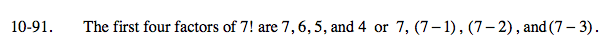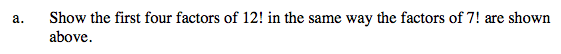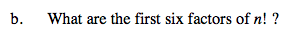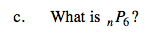### Home > A2C > Chapter 10 > Lesson 10.2.3 > Problem10-91

10-91.
1. The first four factors of 7! are 7, 6, 5, and 4 or 7, (7 − 1), (7 − 2), and(7 − 3). Homework Help ✎

1. Show the first four factors of 12! in the same way the factors of 7! are shown above.

2. What are the first six factors of n!?

3. What is nP6?Begin with 12, (12−1) ... and write out four factors.Write them in the same way you wrote the factors for part (a).Remember the meaning of nP6: choose and arrange 6 items from a collection of n items.

n(n − 1)(n − 2)(n − 3)(n − 4)(n − 5)